## Quiz 6 : Accounting for and Presentation of Property, Plant and Equipment, and Other Noncurrent AssetsLooking for Accounting Homework Help?# Quiz 6 : Accounting for and Presentation of Property, Plant and Equipment, and Other Noncurrent Assets

Compute the cost that should be recorded for the commercial safe: A basket, or lump sum purchase, occurs when multiple noncurrent assets are purchased in a single transaction. The cost of each asset must be calculated and recorded separately. In order to do so, an allocation of the total cost of the purchase is made to each asset based on their individual appraised value on the date of purchase. Compute the fair value of basket: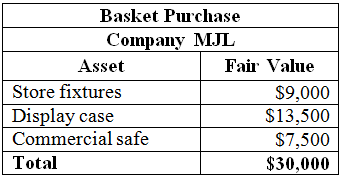Calculate the percent of total for the safe: To calculate the percent of total for the safe, divide the appraised fair value of the safe by the total fair value of the individual assets purchased:Calculate the cost of the commercial safe: To calculate the cost of the commercial safe, multiple the total basket purchase price by the percentage allocation of the safe:Hence, the cost of commercial safe is \$4,500.

Write when the expenditure will be capitalized or expensed: • The capital expenditure should be recognized only, if the item acquired is an asset. The asset should provide an economic benefit to the company beyond the current fiscal year. • If the expenditure is not capitalized, the expenditure should be expensed. When the expenditure is capitalized, there will be a delay in the recognition of the expense by spreading it out over the lifespan of the asset acquired. • Expensing means that that the expenditure will be recognized immediately. Write which of the given expenditures should be capitalized or expensed: • The \$4,200 cost to replace the transmission in a company-owned vehicle should be capitalized. This repair will extend the useful life of the asset. • The \$9,700 cost to develop and register a design patent should be capitalized. The patent will provide an economic benefit to the company beyond the current fiscal year. A patent is an intangible asset, meaning its cost should be amortized over the lifespan of the patent. • The \$24,600 cost to add a security and monitoring system to the company's distribution center should be capitalized because it will add a significant value to the asset. • The \$12,400 cost of annual property insurance on the company's production facility should be expensed. This is a normal cost of doing business and is not an expenditure made to purchase an asset that extends beyond the current fiscal year. • The \$500 cost to repair paint damage on a company-owned vehicle caused by normal wear and tear should be expensed because it will likely not increase the useful life of the asset.

(a) Compute the depreciation expense to be recognized for 2016: Straight-line depreciation expense is a method of calculating the depreciation expense. Under this method, the cost of the asset is spread over the useful life of the asset. Under this method, the depreciation expense is calculated by dividing the net cost (cost minus salvage) with the useful life in years. To calculate the depreciation expense for 2016 for Company GCC's equipment, first calculate the amount to be depreciated, which is Cost - Salvage Value: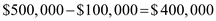Then, calculate annual depreciation expense by dividing the amount to be depreciated by the estimated useful life of the asset:To calculate the equipment's net book value on December 31, 2018, after the third year of the equipment's life, multiply the yearly depreciation expense by the number of years: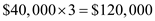Then, subtract the depreciation expense for three years from the cost of the asset: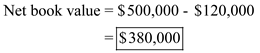(b) Compute the depreciation expense to be recognized for 2018 using double declining balance method: The double declining balance is method is one of the depreciation method used to calculate the depreciation expense. Under this method, double the straight-line rate is used calculate the depreciation expense. To calculate the depreciation expense for the third year of the equipment's life using declining-balance depreciation at twice the straight-line rate, calculate the straight-line rate and double it: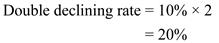Then, calculate the depreciation expense and net book value of the asset at the end of each year: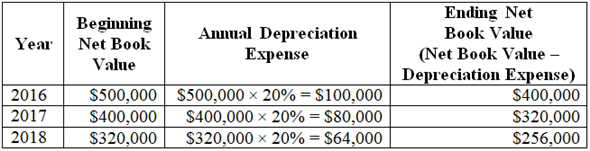Hence, the depreciation expense at December 31, 2008 is \$256,000.